# 语音特征参数MFCC提取过程详解

2014/01/15 17:19

折腾了好几天，看了很多资料，终于把语音特征参数MFCC搞明白了，闲话少说，进入正题。

在语音识别（Speech Recognition）和话者识别（Speaker Recognition）方面，最常用到的语音特征就是梅尔倒谱系数（Mel-scale Frequency Cepstral Coefficients，简称MFCC）。根据人耳听觉机理的研究发现，人耳对不同频率的声波有不同的听觉敏感度。从200Hz到5000Hz的语音信号对语音的清晰度影响对大。两个响度不等的声音作用于人耳时，则响度较高的频率成分的存在会影响到对响度较低的频率成分的感受，使其变得不易察觉，这种现象称为掩蔽效应。由于频率较低的声音在内耳蜗基底膜上行波传递的距离大于频率较高的声音，故一般来说，低音容易掩蔽高音，而高音掩蔽低音较困难。在低频处的声音掩蔽的临界带宽较高频要小。所以，人们从低频到高频这一段频带内按临界带宽的大小由密到疏安排一组带通滤波器，对输入信号进行滤波。将每个带通滤波器输出的信号能量作为信号的基本特征，对此特征经过进一步处理后就可以作为语音的输入特征。由于这种特征不依赖于信号的性质，对输入信号不做任何的假设和限制，又利用了听觉模型的研究成果。因此，这种参数比基于声道模型的LPCC相比具有更好的鲁邦性，更符合人耳的听觉特性，而且当信噪比降低时仍然具有较好的识别性能。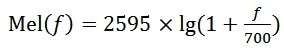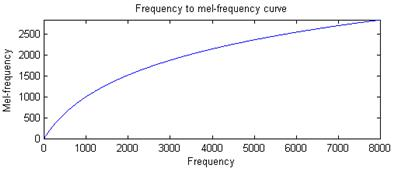基本步骤：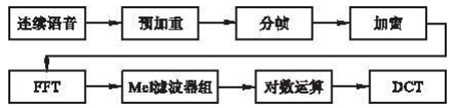1.预加重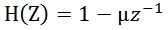(2）

2.分帧

3.加窗（Hamming Window）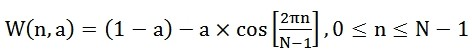(3）

4.快速傅里叶变换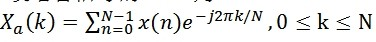(4)

式中x(n)为输入的语音信号，N表示傅里叶变换的点数。

5. 三角带通滤波器

将能量谱通过一组Mel尺度的三角形滤波器组，定义一个有M个滤波器的滤波器组（滤波器的个数和临界            带的个数相近），采用的滤波器为三角滤波器，中心频率为f(m),m=1,2,...,M。M通常取22-26。各                f(m)之间的间隔随着m值的减小而缩小，随着m值的增大而增宽，如图所示：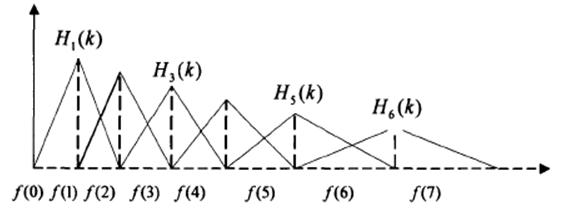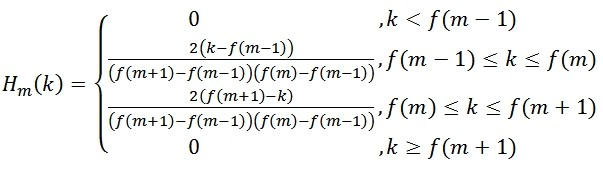(5)

式中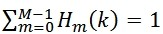6.计算每个滤波器组输出的对数能量为：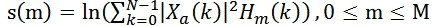(6)

7.经离散余弦变换（DCT）得到MFCC系数：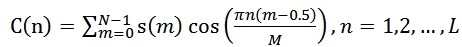(7)

8.对数能量

9.动态差分参数的提取（包括一阶差分和二阶差分）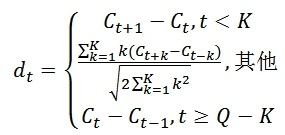(8)

N维MFCC参数（N/3 MFCC系数+ N/3 一阶差分参数+ N/3 二阶差分参数）+帧能量（此项可根据需求替换）

MATLAB实现

[x fs]=wavread('8.wav');
bank=melbankm(24,256,fs,0,0.4,'t');%Mel滤波器的阶数为24，fft变换的长度为256，采样频率为16000Hz
%归一化mel滤波器组系数
bank=full(bank);
bank=bank/max(bank(:));
for k=1:12			%归一化mel滤波器组系数
n=0:23;
dctcoef(k,:)=cos((2*n+1)*k*pi/(2*24));
end
w=1+6*sin(pi*[1:12]./12);%归一化倒谱提升窗口
w=w/max(w);%预加重滤波器
xx=double(x);
xx=filter([1-0.9375],1,xx);%语音信号分帧
xx=enframe(xx,256,80);%对x 256点分为一帧
%计算每帧的MFCC参数
for i=1:size(xx,1)
y=xx(i,:);
s=y'.*hamming(256);
t=abs(fft(s));%fft快速傅立叶变换
t=t.^2;
c1=dctcoef*log(bank*t(1:129));
c2=c1.*w';
m(i,:)=c2';
end
%求取一阶差分系数
dtm=zeros(size(m));
for i=3:size(m,1)-2
dtm(i,:)=-2*m(i-2,:)-m(i-1,:)+m(i+1,:)+2*m(i+2,:);
end
dtm=dtm/3;
%求取二阶差分系数
dtmm=zeros(size(dtm));
for i=3:size(dtm,1)-2
dtmm(i,:)=-2*dtm(i-2,:)-dtm(i-1,:)+dtm(i+1,:)+2*dtm(i+2,:);
end
dtmm=dtmm/3;
%合并mfcc参数和一阶差分mfcc参数
ccc=[m dtm dtmm];
%去除首尾两帧，因为这两帧的一阶差分参数为0
ccc=ccc(3:size(m,1)-2,:);
ccc
% subplot(2,1,1)
% ccc_1=ccc(:,1);
% plot(ccc_1);title('MFCC');ylabel('幅值');
% [h,w]=size(ccc);
% A=size(ccc);
% subplot(212)
% plot([1,w],A);
% xlabel('维数');
% ylabel('幅值');
% title('维数与幅值的关系') 

HTK实现：

运行：
HCopy config 8.wav 8.mfcc

TARGETKIND=MFCC_E_D_A
-目标是MFCC文件，以及energy(E), delta(D),delta-delta(A)
TARGETRATE=100000
-窗间隔为10ms
WINDOWSIZE=250000
-窗长为25ms

ZMEANSOURCE=T
-将来源文件取zero mean,即去掉DC值
USEHAMMING=T
-使用hamming window
PREEMCOEF=0.97
-预加重系数0.97
NUMCHANS=31
-在MEL刻度下等分成31个频带
USEPOWER=F
-不使用c(0)参数### 作者的其它热门文章

9
42 收藏

12 评论
42 收藏
9